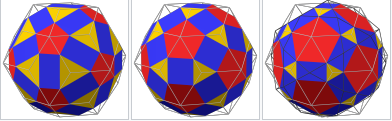# Images . Rhombicosidodecahedron (V=60) from Biscribed Pentakis Dodecahedron for the case of trisection of its 8th-order segments(Variant1)

Generating Elements of mesh modeling the surfaces of polyhedron, its dual image and the coloring of their edges and faces can be found in the applet﻿.
Elements in polyhedron Biscribed Pentakis Dodecahedron(8) : Vertices: V=60. Faces: F=62. (20 equilateral triangles{3} + 30 rectangles{4} + 12 regular pentagons{5}) Edges: E=120. 60+60 - The order of the number of edges in this polyhedron are according to their length. Rhombicosidodecahedron: Type Archimedean solid; Uniform polyhedron Elements F = 62, E = 120, V = 60 (χ = 2) Faces by sides 20{3}+30{4}+12{5}## Faces and Edges of the Biscribed Pentakis Dodecahedron(8) -Rhombicosidodecahedron (n=60)

The elements of the dual to the Biscribed Pentakis Dodecahedron(8): Vertices: V=62 Faces: F=120. 120 scalene triangles {3} Edges: E=180. 60+60+60 - The order of the number of edges in this polyhedron are according to their length. If we assume that two triangles with a common long side lie in the same plane, we get: Vertices: V=62 Faces: F=60. 60 kites{4} Edges: E=120. 60+60 Deltoidal Hexecontahedron : Vertices: 62 = 12 + 20 + 30 Face polygon: 60 kites{4} Edges: 120